Question Pool Circles

# Circles - SAMAGRA Question Pool & Answers | Class 9 English Medium

Kerala Syllabus SAMAGRA SCERT SAMAGRA Question Pool for Class 9 English Medium Maths CirclesQn 1.

AB&CD are two chords in a circle with equal distance from the centre.If AB=4 cm, What is the value of CD?

CD=4 cm

Get Free Study Materials + 1 Week Free Trial of BrainsPrep Class 9 English Medium Tuition

Qn 2.

AB&CD are two chords in a circle with equal distance from the centre.If AB=4 cm, What is the value of CD?

CD=4 cm

Get Free Study Materials + 1 Week Free Trial of BrainsPrep Class 9 English Medium Tuition

Qn 3.

In the figure M is the centre of the circle . AB and CD are parallel chords. The diameter of the circle is 30 c:m ,AB =18 c:m ,CD= 24 c:m

1. Find the distance from the centre to AB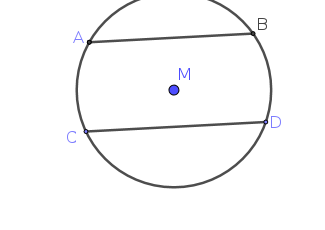2. Find the distance between AB and CD

3. If AB and CD are on the same side of the centre, then what is the distance between them ?

Get Free Study Materials + 1 Week Free Trial of BrainsPrep Class 9 English Medium Tuition

Qn 4.

In the figure M is the centre of the circle AB and CD are two chords .If MQ┴ CD

AB =24 cm and MP= 9 cm

1. Find the radius of the circle ?

2. If MQ=12 cm ,find the length of CQ ?

3. What is the length of the chord CD?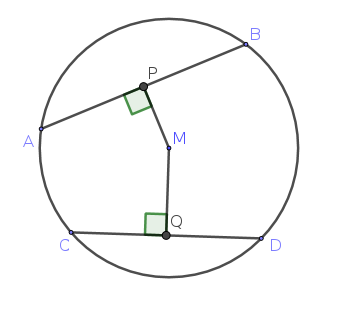Get Free Study Materials + 1 Week Free Trial of BrainsPrep Class 9 English Medium Tuition

Qn 5.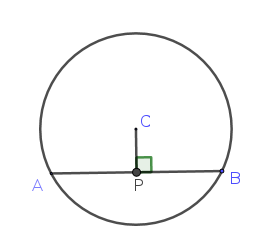In the figure C is the centre of the circle and CP is perpendicular to the chord AB

CP=5 c:m and AB=24 c:m .Then find

1. The length of AP.

2. The radius of the circle

Get Free Study Materials + 1 Week Free Trial of BrainsPrep Class 9 English Medium Tuition

Qn 6.

In the figure AB is diameter and AC is a chord.If AB=70cm and AC=56 cm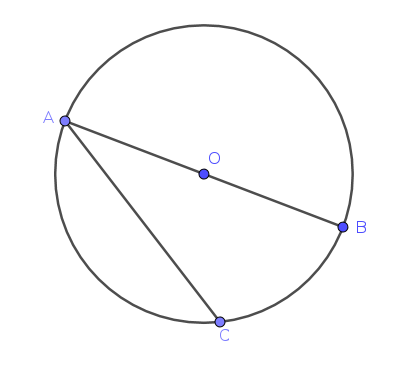1.What is the radius of the circle?

2.Find the distance between the centre and the chord AC?

Get Free Study Materials + 1 Week Free Trial of BrainsPrep Class 9 English Medium Tuition

Qn 7.

Draw a circle of radius 4cm .Draw an equilateral triangle with vertices on the circle?.measure the sides of the triangle?

Get Free Study Materials + 1 Week Free Trial of BrainsPrep Class 9 English Medium Tuition

Qn 8.

1.Draw ∆ABC with AB=6cm,and ∟A=45° ∟B=45° and draw its circumcircle?

1. For drawing the

Get Free Study Materials + 1 Week Free Trial of BrainsPrep Class 9 English Medium Tuition

Qn 9.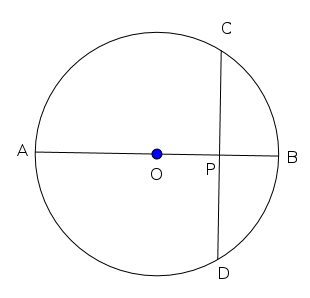In the figure O is the centre of the circle. AB is perpendicular to CD.

PA = 7 cm. , PB = 3 cm.

(a) What is the radius of the circle ?

(b) Find the length of PC.

(c)Find the length of CD.

Get Free Study Materials + 1 Week Free Trial of BrainsPrep Class 9 English Medium Tuition

Qn 10.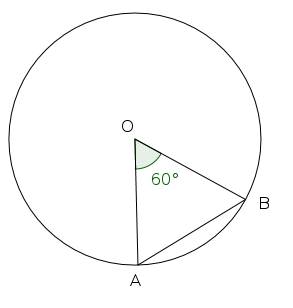In the figure O is the centre of the circle. ∠AOB =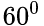, AB = 10 cm.

(a) What is the radius of the circle ?

(b) Find the length of the chord at a distance 7 cm. from the centre

Get Free Study Materials + 1 Week Free Trial of BrainsPrep Class 9 English Medium Tuition

Qn 11.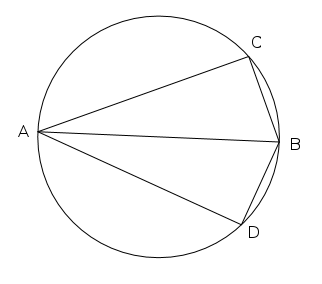In the figure AB is the diameter of the circle. ∠CAB = ∠DAB

(a) What is the measure of ∠ACB ?

(b) If AC = AD prove that BC = BD .

Get Free Study Materials + 1 Week Free Trial of BrainsPrep Class 9 English Medium Tuition

Qn 12.

(a) Draw a line AB of length 2 cm.?

(b)Draw two circles passing through A and B.

(c) Howmany circles can be drwan through A and B ? What is the radius of the smallest amoung them ?

(a) Draws a line AB= 2 cm

Get Free Study Materials + 1 Week Free Trial of BrainsPrep Class 9 English Medium Tuition

Qn 13.

In Figure A is the centre and AD is the perpendicular drawn from centre to chord BC.

a)Which are the equal sides of triangle ABC?

b)  In any circle, prove that the line joining the centre and midpoint of a chord is perpendicular to that chord.

a) AB,